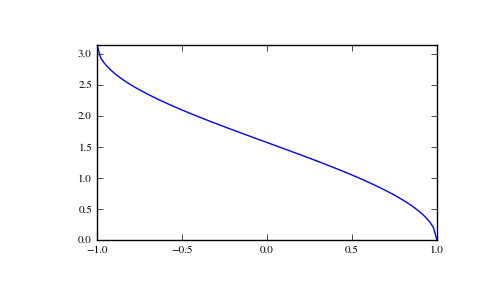# numpy.arccos¶

numpy.arccos(x[, out]) = <ufunc 'arccos'>

Trigonometric inverse cosine, element-wise.

The inverse of cos so that, if y = cos(x), then x = arccos(y).

Parameters : x : array_like x-coordinate on the unit circle. For real arguments, the domain is [-1, 1]. out : ndarray, optional Array of the same shape as a, to store results in. See doc.ufuncs (Section “Output arguments”) for more details. angle : ndarray The angle of the ray intersecting the unit circle at the given x-coordinate in radians [0, pi]. If x is a scalar then a scalar is returned, otherwise an array of the same shape as x is returned.

cos, arctan, arcsin, emath.arccos

Notes

arccos is a multivalued function: for each x there are infinitely many numbers z such that cos(z) = x. The convention is to return the angle z whose real part lies in [0, pi].

For real-valued input data types, arccos always returns real output. For each value that cannot be expressed as a real number or infinity, it yields nan and sets the invalid floating point error flag.

For complex-valued input, arccos is a complex analytic function that has branch cuts [-inf, -1] and [1, inf] and is continuous from above on the former and from below on the latter.

The inverse cos is also known as acos or cos^-1.

References

M. Abramowitz and I.A. Stegun, “Handbook of Mathematical Functions”, 10th printing, 1964, pp. 79. http://www.math.sfu.ca/~cbm/aands/

Examples

We expect the arccos of 1 to be 0, and of -1 to be pi:

```>>> np.arccos([1, -1])
array([ 0.        ,  3.14159265])
```

Plot arccos:

```>>> import matplotlib.pyplot as plt
>>> x = np.linspace(-1, 1, num=100)
>>> plt.plot(x, np.arccos(x))
>>> plt.axis('tight')
>>> plt.show()
```numpy.arcsin

numpy.arctan